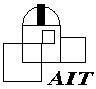## Institut für Astronomie und Astrophysik

### Abteilung Astronomie

Sand 1, D-72076 Tübingen, GermanyKSONE Source code in ksone.pro

### KSONE

Name
```       KSONE
```
Purpose
```       Compute the one-sided Kolmogorov-Smirnov statistic
```
Explanation
```       Returns the Kolmogorov-Smirnov statistic and associated probability for
for an array of data values and a user-supplied cumulative distribution
function (CDF) of a single variable.   Algorithm from the procedure of
the same name in "Numerical Recipes" by Press et al. 2nd edition (1992)
```
Calling Sequence
```       ksone, data, func_name, D, prob, [ /PLOT ]
```
Input Parameters
```       data -  vector of data values, must contain at least 4 elements for the
K-S statistic to be meaningful
func_name - scalar string giving the name of the cumulative distribution
function.    The function must be defined to accept the data
vector as its only input (see example), though keywords may be
passed via the _EXTRA facility.
```
Output Parameters
```       D - floating scalar giving the Kolmogorov-Smirnov statistic.   It
specified the maximum deviation between the cumulative
distribution of the data and the supplied function
prob - floating scalar between 0 and 1 giving the significance level of
the K-S statistic.   Small values of PROB show that the
cumulative distribution function of DATA is significantly
different from FUNC_NAME.
```
Keyword Parameters
```       PLOT - If this keyword is set and non-zero, then KSONE will display a
plot of the CDF of the data with the supplied function
superposed.   The data value where the K-S statistic is
computed (i.e. at the maximum difference between the data CDF
and the function) is indicated by a vertical line.
KSONE accepts the _EXTRA keyword, so that most plot keywords
(e.g. TITLE, XTITLE, XSTYLE) can also be passed to KSONE.
```
Example
```       Determine if a vector created by the RANDOMN function is really
consistent with a Gaussian distribution with unit variance.
The CDF of a Gaussian is the error function except that a factor
of 2 is included in the error function.   So we must create a special
function:
function gauss_cdf, x
return, errorf( x/sqrt(2) )
end
IDL> data = randomn(seed, 50)          ;create data array to be tested
IDL> ksone, abs(data), 'gauss_cdf', D, prob, /PLOT     ;Use K-S test
PROB gives the probability that the null hypothesis (DATA came from a
Gaussian distribution with unit variance) is correct.
```
Note
```       The code for PROB_KS is from the 2nd (1992) edition of Numerical
Recipes which includes a more accurate computation of the K-S
significance for small values of N than the first edition.
```
Procedures Used
```       procedure PROB_KS - computes significance of K-S distribution
```
Revision History
```       Written     W. Landsman                   August, 1992
Accept _EXTRA keywords   W. Landsman      September, 1995
Fixed possible bug in plot display showing position maximum difference
in histogram   M. Fardal/ W. Landsman      March, 1997
Converted to IDL V5.0   W. Landsman   September 1997
Documentation updates   W. Landsman   June 2003
Pass _EXTRA to func_name  M. Fitzgerald    April, 2005
```